Hello Guest it is April 11, 2021, 08:52:24 AM

###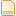Author Topic: How to read/write Analog Inputs and Analog Outputs  (Read 645 times)

0 Members and 1 Guest are viewing this topic.

####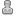SwiftyJ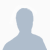•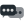85##### Re: How to read/write Analog Inputs and Analog Outputs
« Reply #10 on: May 11, 2020, 08:30:36 AM »
Try putting a 1 in the denominator and numerator column for each of the inputs

####bcoop•48##### Re: How to read/write Analog Inputs and Analog Outputs
« Reply #11 on: May 11, 2020, 09:15:19 AM »
Ha,  that worked!    I guess I never noticed the scaling method implemented,   thank you very much!

so here is what worked to read an Analog Input
1. make an assignment to the Analog Input# under Mach's 'Control Configuration / Analog Inputs'
2. set some scaling values in the numerator and denominator - for no scaling factor set to 1 & 1
3. in a script use      local value = mc.mcAnalogInputRead(inst, # )  where # is 0-63

Thanks to everyone

Bob
Bob

####jbuehn•68##### Re: How to read/write Analog Inputs and Analog Outputs
« Reply #12 on: May 11, 2020, 12:14:15 PM »
Craig, I was looking in build 4481 at the analog API functions.

####smurph•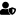•1,373
• "That there... that's an RV."##### Re: How to read/write Analog Inputs and Analog Outputs
« Reply #13 on: May 12, 2020, 12:00:46 AM »
As stated above, you can access the analogs as registers as they come in from the plugin using the register read/write functions.  This will let you access a raw ADC or DAC value, usually something along the lines of 0 to 1023 (10 bit ADC/DAC) or 0 to 4095 (12 bit ADC/DAC), etc...  Mapping these registers to an analog input give you the capability of working with voltages instead of raw ADC/DAC values, if required.

For example, say you have a 12 bit DAC output with a voltage range of 0 to 10v represented by the scale of 0 to 4095, 0 being 0 volts and 4095 being 10 volts:

Set the numerator to the total voltage swing (0 to 10 = 10 volts total) and the denominator equal to the total capacity of the DAC bits.  12 bits == 4096.  Now you can use the mcAnalogOutputWrite() function with volts!

rc = mc.mcAnalogOutputWrite(inst, 1, 7.5) -- Set analog output 1 to 7.5 volts.
rc = mc.mcAnalogOutputWrite(inst, 1, 2) -- Set analog output 1 to 2 volts.

What if you have a bi-polar -10 to +10 volt 12 bit DAC that you want to set?

Set the numerator to the total voltage swing (-10 to +10 = 20 volts total) and the denominator equal to the total capacity of the DAC bits.  12 bits == 4096.  Most bi-polar DACs have mid scale as 0 volts, so set the offset to half of the denominator, 2048.

rc = mc.mcAnalogOutputWrite(inst, 1, -10) -- Set analog output 1 to -10 volts.  Raw DAC value will be 0.
rc = mc.mcAnalogOutputWrite(inst, 1, 0) -- Set analog output 1 to 0 volts.  Raw DAC value will be 2048.
rc = mc.mcAnalogOutputWrite(inst, 1, 10) -- Set analog output 1 to +10 volts.  Raw DAC value will be 4096.

Steve

####bcoop•48##### Re: How to read/write Analog Inputs and Analog Outputs
« Reply #14 on: May 12, 2020, 05:56:30 AM »
Thank you Steve for the examples on using the numerator, denominator, & offset   - very helpful.

Bob

####bcoop•48##### Re: How to read/write Analog Inputs and Analog Outputs
« Reply #15 on: May 14, 2020, 06:30:06 PM »
one more analog question, how do I assign an AnalogInput  or Analogoutput to a to a dro or textbox. to display the value?
Bob

####smurph••1,373
• "That there... that's an RV."##### Re: How to read/write Analog Inputs and Analog Outputs
« Reply #16 on: May 15, 2020, 04:13:31 AM »
I haven't got that implemented yet.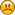Steve

####bcoop•48##### Re: How to read/write Analog Inputs and Analog Outputs
« Reply #17 on: May 15, 2020, 08:49:42 AM »
Ok Steve, good to know,   I  just made the assignment via the PLC script.

all the best
Bob
Bob